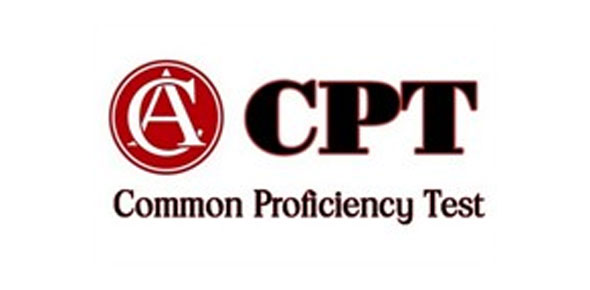# CPT 3 Quiz Review A

Approved & Edited by ProProfs Editorial Team
At ProProfs Quizzes, our dedicated in-house team of experts takes pride in their work. With a sharp eye for detail, they meticulously review each quiz. This ensures that every quiz, taken by over 100 million users, meets our standards of accuracy, clarity, and engagement.
| Written by Mrsrowland
M
Mrsrowland
Community Contributor
Quizzes Created: 3 | Total Attempts: 1,941
Questions: 9 | Attempts: 183SettingsCombine like terms and use the order of operations to simplify algebraic expressions.

• 1.

### 3x + 1 + 8x + 9

• A.

12x + 10

• B.

5x + 8

• C.

11x + 11

• D.

11x + 10

D. 11x + 10
Explanation
The given expression is a sum of two terms: 3x + 1 and 8x + 9. To simplify this expression, we combine like terms by adding the coefficients of x and the constants separately. The coefficient of x in the first term is 3 and in the second term is 8, so the sum of the coefficients is 3 + 8 = 11. Similarly, the constant term in the first term is 1 and in the second term is 9, so the sum of the constants is 1 + 9 = 10. Therefore, the simplified expression is 11x + 10.

Rate this question:

• 2.

### 2x + 5y - 7x + 8y

• A.

9x + 13y

• B.

5x + 3y

• C.

-5x + 3y

• D.

-5x + 13y

D. -5x + 13y
Explanation
The given expression is a combination of like terms. By combining the terms with the same variable, we can simplify the expression. In this case, combining the x terms (-7x and 2x) gives us -5x, and combining the y terms (5y and 8y) gives us 13y. Therefore, the simplified expression is -5x + 13y.

Rate this question:

• 3.

### 4(x + 8) - 9

• A.

4x + 32

• B.

4x + 23

• C.

12x - 9

• D.

4x + 24

B. 4x + 23
Explanation
The given expression is 4(x + 8) - 9. To simplify it, we first distribute the 4 to both terms inside the parentheses, resulting in 4x + 32. Then, we subtract 9 from this expression, giving us the final simplified form of 4x + 23.

Rate this question:

• 4.

### -3(x + y) + 5(x - y)

• A.

• B.

-2x + 2y

• C.

8x - 8y

• D.

2x - 8y

D. 2x - 8y
Explanation
The given expression is a combination of two terms: -3(x + y) and 5(x - y). To simplify this expression, we can distribute the -3 and 5 to the terms inside the parentheses. This gives us -3x - 3y + 5x - 5y. We can then combine like terms by adding the x terms and the y terms separately. This gives us -3x + 5x - 3y - 5y, which simplifies to 2x - 8y. Therefore, the correct answer is 2x - 8y.

Rate this question:

• 5.

### A + b = b + a is an example of the property called ______________________________________________________________________

c +
commutative
commutative property
Explanation
The given equation, a + b = b + a, demonstrates the commutative property of addition. This property states that the order in which numbers are added does not affect the result. In other words, when adding two numbers, switching their positions will not change the sum. This property is applicable to the addition of any real numbers.

Rate this question:

• 6.

### 9( x + 3) = 9(x) + (9)(3) is an example of what property? ____________________________________________________

distributive
distributive property
Explanation
The given equation 9(x + 3) = 9(x) + (9)(3) demonstrates the distributive property. This property states that when multiplying a number by a sum, you can distribute the multiplication to each term inside the parentheses. In this case, the 9 is being multiplied by the sum of x and 3, and the equation shows that you can distribute the 9 to both x and 3 separately.

Rate this question:

• 7.

### What number is known as the multiplicative identity? ____________________

1
one
One
Explanation
The number that is known as the multiplicative identity is 1. In multiplication, any number multiplied by 1 will always result in the same number. Therefore, 1 acts as the identity element for multiplication, as it leaves other numbers unchanged when multiplied by them. The words "one" and "One" are also correct representations of the number 1.

Rate this question:

• 8.

### 0 • x = _____________

0
Explanation
When any number is multiplied by zero, the result is always zero. In this equation, when zero is multiplied by any number (in this case, x), the result will always be zero. Therefore, the answer to the equation 0 * x is 0.

Rate this question:

• 9.

### Ab(c) = (ab)c is an example of what property? __________________________________

Associative Property of Multiplication
associative property of multiplication
assoc. prop. of mult.
A x
associative property
associative
Explanation
The equation ab(c) = (ab)c demonstrates the Associative Property of Multiplication. This property states that the grouping of factors in a multiplication expression does not affect the result. In this case, the factors a, b, and c can be multiplied in any order and the product will remain the same.

Rate this question:

Related TopicsBack to top
×

Wait!
Here's an interesting quiz for you.